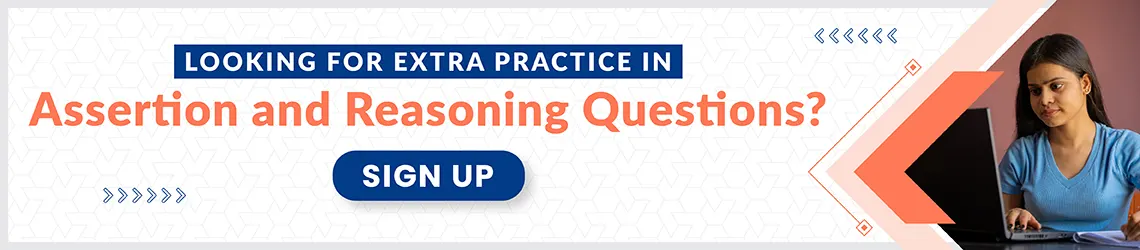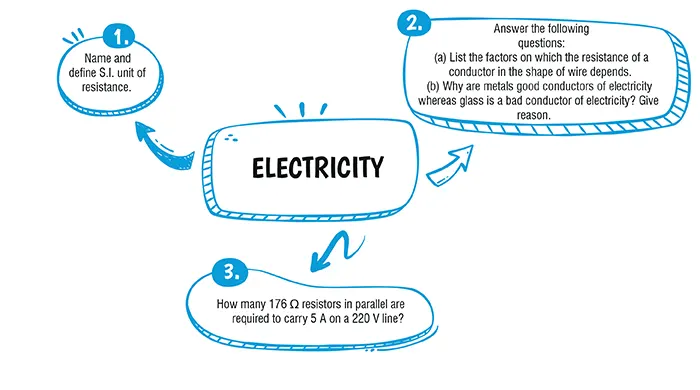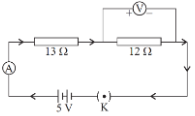Electricity

# Class 10 Science Chapter 11 Electricity Important QuestionsHere are some critical Chapter 11 Electricity Problems for Class 10 Science. These inquiries are intended to aid students in studying for and performing well on the CBSE Class 10 Science Examination 2023–24. Students can clear up their concerns and be ready for the exams by practising different types of questions. By answering these questions, you'll increase your confidence while also sharpening your problem-solving abilities

## Introduction

Chapter 11 Electricity covers Electric Current and Circuit, Electric Potential and Potential Difference, Circuit Diagram, Ohm's Law, Factors on which the Resistance of a Conductor Depends, Resistance of a System of Resistors, Heating Effect of Electric Current and Electric Power.### Class 10 Electricity  Important Questions and Answers

#### Q1. Two resistances are connected in series as shown in the diagram. The potential difference across 12 Ω resistor will be :#### (a) 6 V(b) 2.4 V(c) 2.8 V(d) 12 V

Ans. (b)

Explanation:
Using Ohm’s law, Current,
I = V/R
= 5/ (13+12)
= 5/25
= 0.2 A
So current flowing in 12 Ω resistance = 0.2 A
The potential difference across 12
= IR = 0.2× 12
= 2.4 V

#### (a) 16.8 V  (b) 17.1 V (c) 11.3 V(d) 15.9 V

Ans. (d)

Explanation:
E = V - Ir
V = E + Ir
$$=10+\begin{pmatrix} \frac{150-10}{18+0.8}×0.8\end{pmatrix}$$
= 15.9 V

#### Q3. Name and define S.I. unit of resistance.

Explanation:
The S.I. The unit of resistance is ohm (Ω).The resistance of a conductor is said to be 1 ohm if 1 ampere current flows through it when a potential difference of 1 volt is applied across the ends of the conductor.
$$1 ohm=\frac{1 volt}{1 ampere}$$

#### Q4. Answer the following questions:(a) List the factors on which the resistance of a conductor in the shape of wire depends.(b) Why are metals good conductors of electricity whereas glass is a bad conductor of electricity? Give a reason.

Explanation:
Resistance of a conductor depends directly on its length and is inversely proportional to the area of cross-section.
(b) Metals have free electrons and they move and conduct electricity, whereas glass does not have free electrons and charges to flow as it is an insulator.

#### Q5. How many 176 Ω resistors in parallel are required to carry 5 A on a 220 V line?

Explanation:
Here, Potential difference, V = 220 V, Current, I = 5 A
∴ Resistance,
R =$$\frac{V}{I}$$
=$$\frac{220}{5}= 44 \varOmega$$
Let the number of 176 Ω resistors to be connected in parallel to give an equivalent resistance of 44 Ω be x.
Equivalent resistance of ‘x’ 176 Ω resistance connected
In parallel is
$$\frac{176}{x}\varOmega$$
But,$$\frac{176}{x}=44$$
$$\therefore X=\frac{176}{44}=4$$
Thus,  4 ressisters of 176 Ω each should be connected in parallel.#### CBSE Class 10 Science Chapter wise Important Questions

Chapter No. Chapter Name
Chapter 1 Chemical Reactions and Equations
Chapter 2 Acid, Bases and Salts
Chapter 3 Metals and Non-Metals
Chapter 4 Carbon and its Compounds
Chapter 5 Life Processes
Chapter 6 Control and Coordination
Chapter 7 How do Organisms Reproduce
Chapter 8 Heredity
Chapter 9 Light : Reflection and Refraction
Chapter 10 The Human Eye and the Colourful world
Chapter 11 Electricity
Chapter 12 Magnetic Effects of Electric Current
Chapter 13 Our Environment

#### Conclusion

oswal.io offers a thorough set of questions for learning the topic in a better way if you're looking to further practise and improve your grasp of the concepts covered in the chapter.

#### Q1 : Define electric current.

Ans: Electric current is the amount of charge flowing through a particular area in unit time.

#### Q2: Define one ampere.

Ans:  One ampere is constituted by the flow of one coulomb of charge per second.
1 A = 1 C s-1

#### Q3 : Name a device that you can use to maintain a potential difference between the ends of a conductor. Explain the process by which this device does so.

Ans: A cell or a battery can be used to maintain a potential difference between the ends of a conductor. The chemical reaction within a cell generates the potential difference across the terminals of the cell, even when no current is drawn from it. When it is connected to a conductor, it produces electric current and maintains the potential difference across the ends of the conductor.

#### Q4 : State Ohm's law of electricity.

Ans: It states that the potential difference V, across the ends of a given metallic wire in an electric circuit is directly proportional to the current flowing through it, provided its temperature remains the same. Mathematically,
V ∝ I
V = RI
where R is the resistance of the conductor.

#### Q5 : (a) Why is tungsten used for making bulb filaments of incandescent lamps?(b) Name any two electric devices based on heating effect of electric current

Ans: (a) (i) Tungsten is a strong metal and has a high melting point (3380°C).
(ii) It emits light at high temperatures (about 2500°C).
(b) Electric laundry iron and electric heater are based on the heating effect of electric current.

###### Copyright 2022 OSWAL PUBLISHERS Simplifying ExamsPhone:  (+91) 78959 87722
Mail: support@oswalpublishers.in